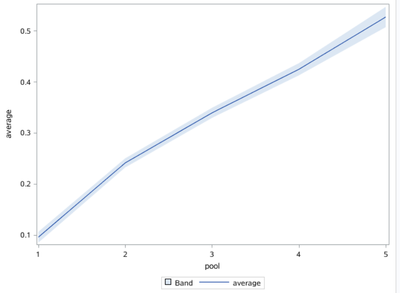## how to create multiple lines plot

 pool count average upper lower 1 2068 0.0953 0.1059 0.0846 2 6120 0.2408 0.2498 0.2319 3 6215 0.3390 0.3489 0.3291 4 4625 0.4244 0.4364 0.4125 5 1695 0.5268 0.5468 0.5069

Hello everyone, know someone how to easily get multiple lines plot from these data?
Upper and lower are confidence interval, average is mean. Count is not relevant and pool is some group.

I'll be glad for any help.

1 ACCEPTED SOLUTION

Accepted Solutions

## Re: how to create multiple lines plot

UNTESTED CODE

``````proc sgplot data=have;
series x=pool y=average;
series x=pool y=upper;
series x=pool y=lower;
run;``````

If you want tested code (and this is always a good idea anyway, @matus2711 ) provide the data as SAS data step code (instructions) and not as a screen capture, something you should just do automatically, every time you ask a question; and from now on we will expect data in this form and not as screen captures.

--
Paige Miller
6 REPLIES 6

## Re: how to create multiple lines plot

UNTESTED CODE

``````proc sgplot data=have;
series x=pool y=average;
series x=pool y=upper;
series x=pool y=lower;
run;``````

If you want tested code (and this is always a good idea anyway, @matus2711 ) provide the data as SAS data step code (instructions) and not as a screen capture, something you should just do automatically, every time you ask a question; and from now on we will expect data in this form and not as screen captures.

--
Paige Miller

## Re: how to create multiple lines plot

Usually, when someone wants a line plot there is an X (indep) and a Y variable (response). What variable do you want to use as X?

For these data, you can create a set of lines that show the average and CI for each group. This kind of plot is sometimes called a "forest plot."  It can be used to compare means of groups or to show odds ratios.

``````data Have;
input pool	count	average	upper	lower;
datalines;
1	2068	0.0953	0.1059	0.0846
2	6120	0.2408	0.2498	0.2319
3	6215	0.3390	0.3489	0.3291
4	4625	0.4244	0.4364	0.4125
5	1695	0.5268	0.5468	0.5069
;

proc sgplot data=Have;
scatter x=pool y=average / yerrorlower=lower yerrorupper=upper;
run;``````

If you want a different plot, please specify or draw it on paper and upload a picture.

--
Paige Miller

## Re: how to create multiple lines plot

To expand on Paige's gracious response, some graphical experts discourage connecting groups with line segments. There is no "Group=1.5" or "Group=2.8", so some folks argue that it is misleading to plot line segments.

## Re: how to create multiple lines plot

@Rick_SAS wrote:

To expand on Paige's gracious response, some graphical experts discourage connecting groups with line segments. There is no "Group=1.5" or "Group=2.8", so some folks argue that it is misleading to plot line segments.

A good point from @Rick_SAS , it is a very common thing for people to treat groups as continuous in their plots, and (ideally) is should be a much less common situation.

--
Paige MillerKsharp
Super User

## Re: how to create multiple lines plot

``````proc sgplot data=have;
band x=pool upper=upper lower=lower/transpancy=0.5;
series x=pool y=average;
run;``````Discussion stats
• 6 replies
• 882 views
• 8 likes
• 4 in conversation